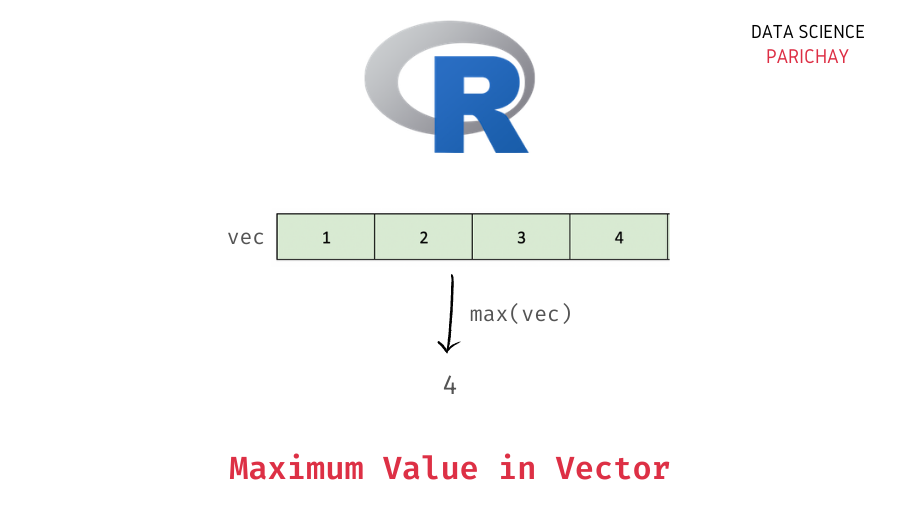# Get the Maximum value in an R Vector

A vector is a one-dimensional data structure used to store data of the same type in R. Numeric vectors are commonly used to store a sequence of numbers. In this tutorial, we will look at how to get the maximum value in an R vector with the help of some examples.

## How to get the max value in a vector in R?

You can use the R `max()` function to get the maximum value in a vector. Pass the vector as an argument to the function. The following is the syntax –

```# get the max value in a vector
max(x, na.rm=FALSE)```

The following are the arguments that you can give to the `max()` function in R.

• x – The vector for which you want to compute the max value.
• na.rm – (Optional argument) Indicates whether to remove missing values before computing the maximum. It is `FALSE` by default.

The function returns the max value in the passed vector.

## Examples

Let’s look at some examples of using the above method to get the max value in a vector.

### Maximum value in a numeric vector

Let’s create a vector of numbers (and without any `NA` values) and apply the `max()` function.

```# create a vector of numbers
vec <- c(1, 3, 5, 4)
# max value in vector
max(vec)```

Output:

`5`

We get 5 as the max value in the above vector, which is the correct answer.

📚 Data Science Programs By Skill Level

Introductory

Intermediate ⭐⭐⭐

🔎 Find Data Science Programs 👨‍💻 111,889 already enrolled

Disclaimer: Data Science Parichay is reader supported. When you purchase a course through a link on this site, we may earn a small commission at no additional cost to you. Earned commissions help support this website and its team of writers.

What would happen if there are some `NA` present values in the vector?

Let’s find out.

First, we will create a vector with some `NA` values and then apply the `max()` function without any additional arguments.

```# create a vector with NA values
vec <- c(1, 3, NA, 5, NA, 4, NA)
# max value in the vector
max(vec)```

Output:

`<NA>`

You can see that we get `NA` as the output. This is because comparing a value with NA results in an `NA` in R.

### Maximum in a vector with `NA` values

You can pass `TRUE` to the `na.rm` parameter of the `max()` function to exclude missing values when computing the maximum value in a vector.

```# create a vector with NA values
vec <- c(1, 3, NA, 5, NA, 4, NA, NA)
# max value in the vector
max(vec, na.rm=TRUE)```

Output:

`5`

Now we get the maximum value in the above vector as 5.

### Maximum value in a character vector

The `max()` function also works similarly on a vector of characters. For example, let’s see what we get on applying the `max()` function on a vector of characters.

```# create a vector of chracters
vec <- c("a", "b", "c", "d")
# max value in the vector
max(vec)```

Output:

`'d'`

Here, we get ‘d’ as the maximum value in the above vector which contains the values ‘a’, ‘b’, ‘c’, and ‘d’.

You might also be interested in –

•